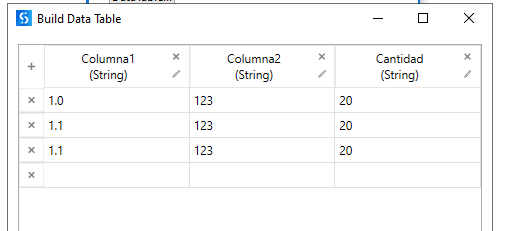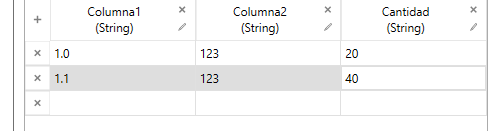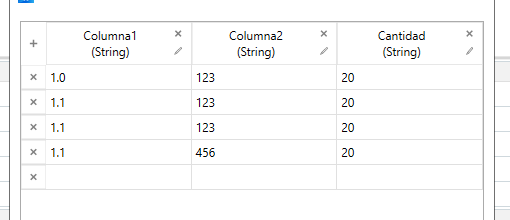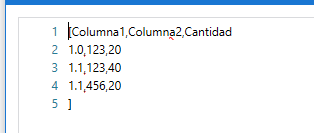# In DataTable find equals rows and unify them and sum values of one column

for example in this dtunify rows that are the same like columna1 1.1 values and columna2 123 and SUM col3 “cantidad”

so the result should behow to do it?

Regards
Sudharsan

Hi @amy93amanda ,

There are many posts in here, which provides the relevant solution to your requirement as it is a Group By and Sum case. One of the posts is mentioned below :

Also, Find below Detailed methods on Grouping :

Hi @amy93amanda,

Use the below expression in assign activity:

(From d In Dt.AsEnumerable Group d By k=d(“Columna1”).toString.Trim Into grp=Group Let sumVal= grp.Sum(Function (x) CDbl(x(“Cantidad”).toString)) let rows=grp.FirstOrDefault Let ra = New Object(){k, rows(“Columna2”).tostring, sumVal} Select Dt.Rows.Add(ra)).CopyToDataTable

1 Like

Hi @amy93amanda ,

First create a dt_Grouped datatable variable and clone with input table as below in assign,
dt_Grouped = dt_Input.Clone()

Then in second assign use below linq,

``````dt_Grouped = (From row in dt_Input.AsEnumerable
Group row by k=row("Columna1").ToString.Trim Into grp=Group
Let result = New Object(){grp(0)("Columna1").ToString,grp(0)("Columna2").ToString,total}
``````

Hope this may help you3 Likes

thank so much, mark as solution but i implement because actually need multiple columns to group by beside the first thanks!!

for this(From d In tabla.AsEnumerable Group d By k=d(“Columna1”).toString.Trim , k2=d(“Columna2”).toString.trim Into grp=Group Let sumVal= grp.Sum(Function (x) CDbl(x(“Cantidad”).toString)) let rows=grp.FirstOrDefault Let ra = New Object(){k, rows(“Columna2”).tostring, sumVal} Select tabla.Rows.Add(ra)).CopyToDataTablethanks also to the rest to find other threads an info to learn!!

2 Likes

also works!

This topic was automatically closed 3 days after the last reply. New replies are no longer allowed.